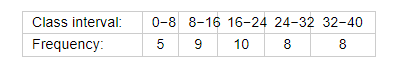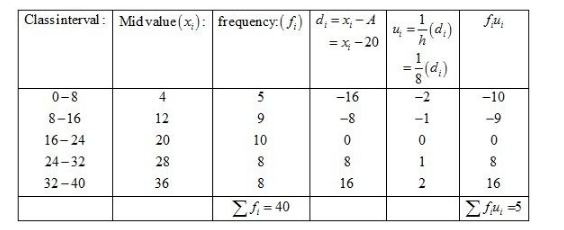# Find the mean of each of the following frequency distributions :

Question:

Find the mean of each of the following frequency distributions :Solution:

Let the assumed mean be A = 20 and h = 8.We know that mean, $\bar{X}=A+h\left(\frac{1}{N} \sum f_{i} u_{i}\right)$

Now, we have $N=\sum f_{i}=40, \sum f_{i} u_{i}=5, h=8$ and $A=20$.

Putting the values in the above formula, we have

$\bar{X}=A+h\left(\frac{1}{N} \sum f_{i} u_{i}\right)$

$=20+8\left(\frac{1}{40} \times(5)\right)$

$=20+\frac{40}{40}$

$=20+1$

$=21$

Hence, the mean is 21.